# The graph of the function

Definition: the Graph of a function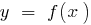is the set of all points in the coordinate plane with the coordinates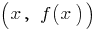where the first coordinateruns the whole of the domain of the function(and the second coordinate is the corresponding value of the functionat the point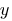).

## Graphs of elementary functions

###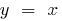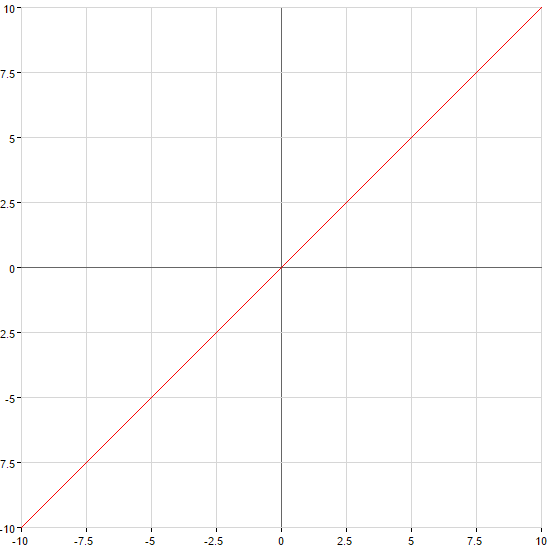###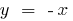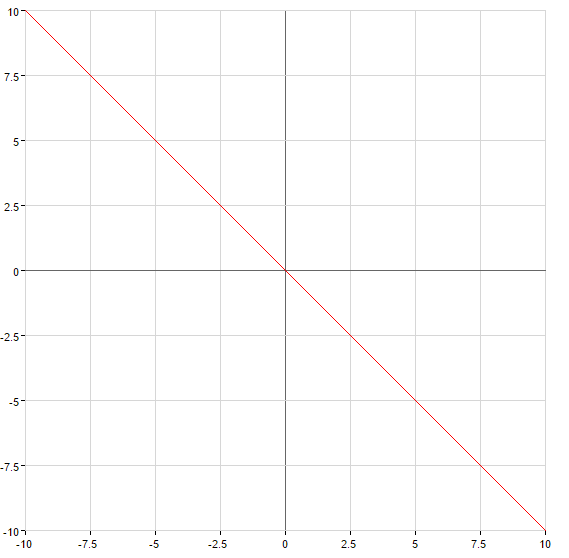###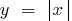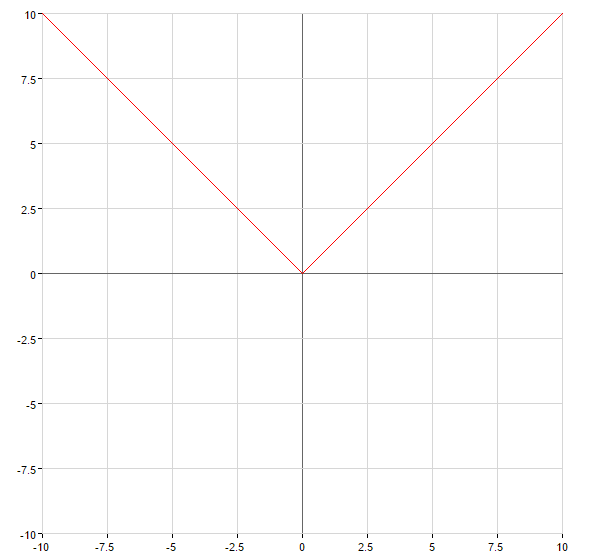###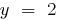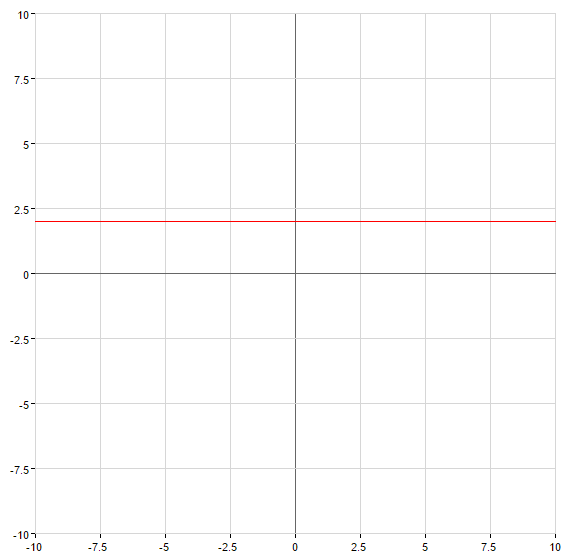###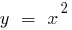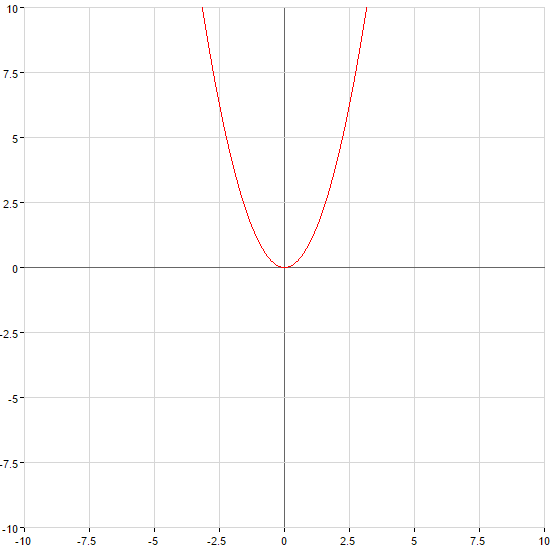###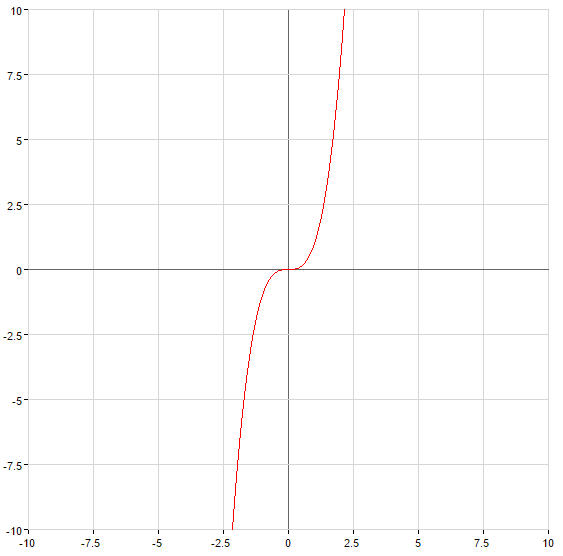###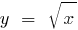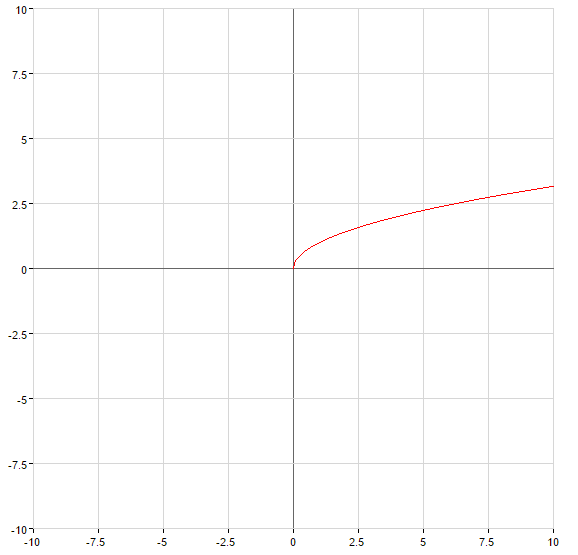###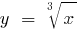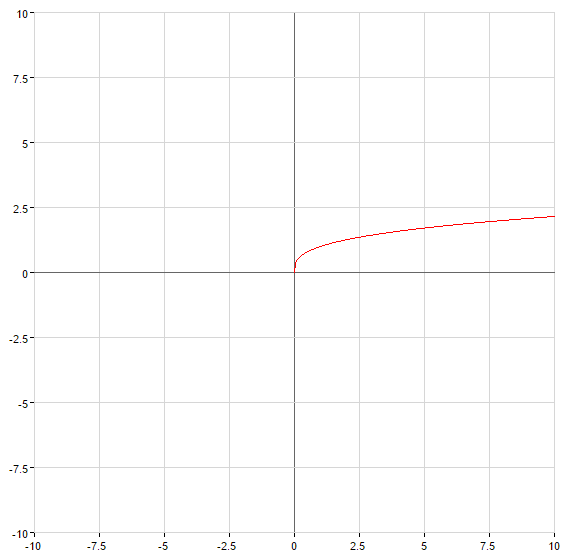###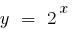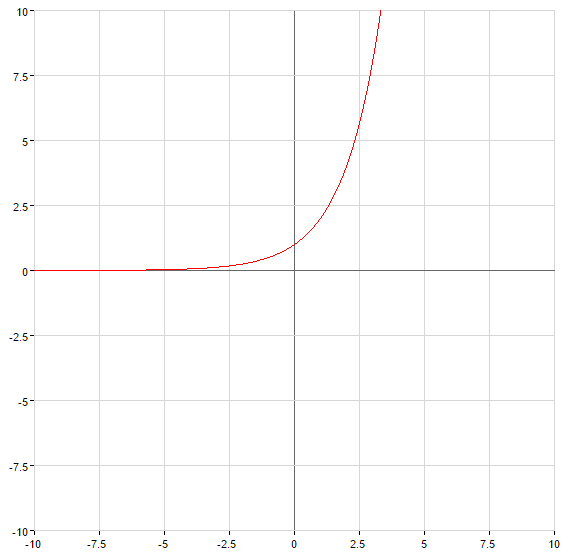###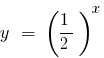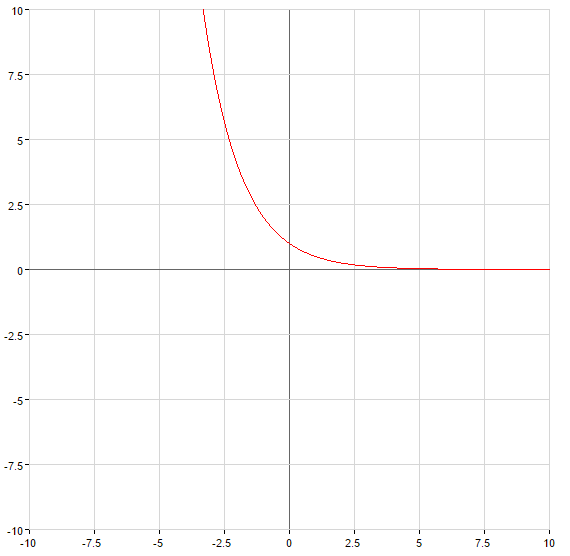###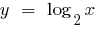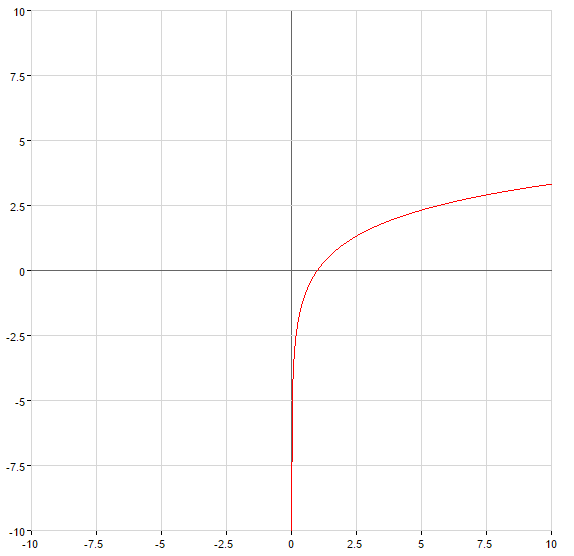###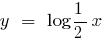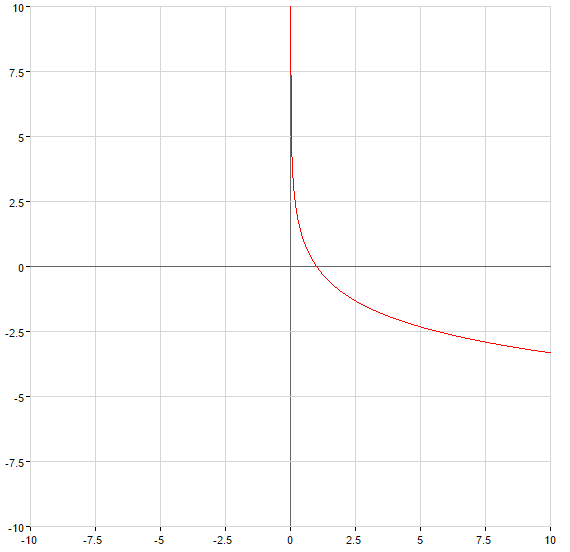Tags:
Chapter:
Versions in other languages:
Share with friends: